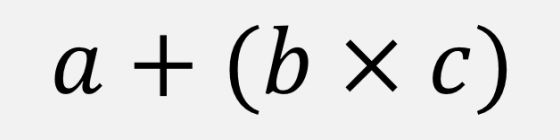시간 제한 메모리 제한 제출 정답 맞은 사람 정답 비율
1 초 512 MB 220 50 33 25.000%

문제The correspondence among the operators and the operands can be clarified using parentheses. In a C program, for example, the expression a-b-c can be clarified as (a-b)-c since the minus operator is left associative.

The parentheses can be used to override the default precedences and associativities of operators. For instance, in the expression a-(b-c), the left associativity of the minus operator is overridden by the parentheses.

A novice C programmer Dennis has been stressed too much in remembering the operator precedences and associativities. Therefore, he made a new language namely ICPC (I can parenthesize C), in which the operator-operand correspondence should be clarified fully using parentheses; except for this, all the other features are same as C. For instance, one should write (a-b)-c instead of a-b-c and a+(b*c) rather than a+b*c.

However, the usage of the parentheses can be too much in some cases. For the expression a-b-c, it is enough to write

(a-b)-c

but one can write it as

(a-(b))-c

or as

((a-b)-c)

where the pairs of parentheses underlined are superfluous.

Dennis wants to convert the C expression into the ICPC expression, in which the pairs of parentheses should be used exactly as needed. You have to help Dennis. For simplicity, you can assume that the input C expression contains only five binary arithmetic operators (+, -, *, /, and %), left and right parentheses ( and ), and single-lowercase alphabet operands. Given such a C expression, write a program to determine whether it is an ICPC expression or not.

If the expression is not an error in ICPC, then it should not be an error in C. Once it is not an error in C, the usage of parentheses should be checked to determine whether it is a proper expression in ICPC or not. If the expression is not properly parenthesized, i.e., the number of parentheses is not exact as needed, then it is considered improper.

Beware that some of the input C expressions may be erroneous originally. For instance, a%/b is an error since it requires one more operand between % and / to be valid. As another example, a b + c is also an error since it requires one more operator between a and b.

입력

Your program is to read from standard input. The input consists of a single line containing a C expression. The expression is a string of single-lowercase alphabets, special symbols including left and right parentheses and five binary arithmetic operators (+, -, *, /, and %), and spaces. The input line contains at least one operand. The length of the input line (the number of characters in it) is no more than 1000 including the spaces and the single newline character at the end.

출력

Your program is to write to standard output. Print exactly one line. The line should contain one of the following words: error, proper, and improper. Print error if the input C expression is erroneous. Once it is not an error, print proper or improper depending on the parenthesized status of the expression: print proper if it is parenthesized properly with the exact number of parentheses needed for ICPC, and print improper otherwise.

a + a

proper

(b+( a+c )) + b

proper

c + ((b) + a)

improper

c+(a%/b)

error

x + ((y + z)

error

a b + (c + b)

error

x + y + z

improper